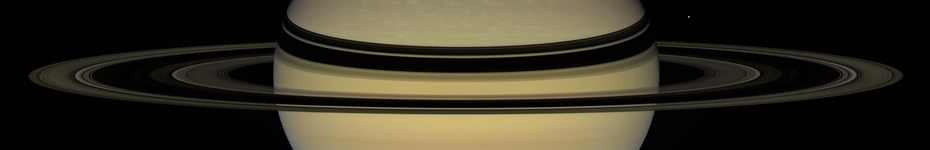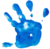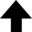My question is this: What kind of curve would be described by the planet, supposing the force of the attraction toward the Sun to be reciprocal to the square of the distance from it?Edmond Halley, during a visit to Isaac Newton in 1684

## Elliptical Orbits from Universal Gravitation

Kepler's three laws of orbital motion can be viewed as concise descriptions of how celestial objects move. On the other hand, they could also be looked upon as rather empty observations that provide little or no insight into why a given orbit is physically allowed. Edmond Halley and his colleagues, reflecting on this, suspected that Kepler's law might emerge from some deeper truth.

### Kepler's First Law: The Law of OrbitsThe above quote contains a rather esoteric-sounding question with a surprisingly simple answer: an ellipse. Halley was referring to Kepler's first law, but how can this be proven? Halley and his colleagues were wondering the same thing.

Isaac Newton's original proof to Halley's question (a treatise of nine pages) was written in the traditional language of Euclidean geometry; here we will use the modern—and more efficient—method of calculus. So, the problem:

Prove that the orbit of a satellite is elliptical if the gravitational force is modeled as the inverse-square of the distance between two bodies of sufficient mass.

As usual, we begin with Newton's second law, $\mathbf{F} = m \mathbf{a}$. The magnitude of the gravitational force is $G M m / r^2$ and the direction is radially inward, which we define as negative (attractive). We set this force equal to $m a$ and include the centripetal acceleration $r \omega^2$ since the mass $m$ is exhibiting circular motion:

 $\displaystyle{ -G \frac{M m}{r^2} = m \frac{\mathrm{d}^2 r}{\mathrm{d} t^2} - m r \omega^2 \text{.} }$ (

Before we are able to integrate Equation (1), we'll need to eliminate $\omega$. Why? Johannes Kepler, through a series of observations in 1605, found that the orbit of Mars was elliptical, meaning that the angular velocity $\omega$ of this planet (and all others) varied as it progressed in its yearly cycle around the Sun. We can accomplish this task by representing $\omega$ in terms of a quantity of angular momentum $L = I \omega = m r^2 \omega$, which is constant. For simplicity we'll also think of the mass $m$ as a point particle (we wrote $m r^2$ for the moment of inertia; a redundant factor of $m$ will cancel). Substituting and rearranging, we get

 \displaystyle{ \begin{align*} \frac{\mathrm{d}^2 r}{\mathrm{d} t^2} & = -\frac{G M}{r^2} + r \left( \frac{L}{m r^2} \right)^2 \\ & = -\frac{G M}{r^2} + \frac{L^2}{m^2 r^3} \text{.} \end{align*} } (

Now make the change of variable $u = 1/r$ in the angular momentum of the point mass. The reader should note that this change of variable is arbitrary in the strict sense that it yields a differential equation that is relatively easy to solve, and whose solution permits a straightforward interpretation. (Similar to a wise choice of coordinate system, a wise choice of integration variable will make our task easier.) We find

 $\displaystyle{ L = m r^2 \omega = \frac{m}{u^2} \frac{\mathrm{d} \theta}{\mathrm{d} t} \text{,} }$ (

keeping in mind that physics is invariant under a transformation of coordinates. In other words, there is no necessity in attaching a physical meaning to the change of variable, as the outcome will be the same regardless of which coordinates we choose. Next, rearrange to get our operator:

 $\displaystyle{ \frac{\mathrm{d}}{\mathrm{d} t} = \frac{L u^2}{m} \frac{\mathrm{d}}{\mathrm{d} \theta} \text{.} }$ (

Equation (4), operating on the coordinate $u$, can be used to specify the change in position of the point mass as a function of time:

 $\displaystyle{ \frac{\mathrm{d} r}{\mathrm{d} t} = \frac{\mathrm{d}}{\mathrm{d} t} \frac{1}{u} = -\frac{1}{u^2} \frac{\mathrm{d} u}{\mathrm{d} t} = -\frac{L}{m} \frac{\mathrm{d} u}{\mathrm{d} \theta} \text{.} }$ (

We get, by extension,

 $\displaystyle{ \frac{\mathrm{d}^2 r}{\mathrm{d} t^2} = -\frac{L^2 u^2}{m^2} \frac{\mathrm{d}^2 u}{\mathrm{d} \theta^2} \text{.} }$ (

Equating (6) to Equation (2) and not forgetting that $u = 1/r$, we find

 $\displaystyle{ \frac{\mathrm{d}^2 u}{\mathrm{d} \theta^2} + u = \frac{G M m^2}{L^2} \text{,} }$ (

which is the equation of motion to be solved. The solution,

 $\displaystyle{ u = \frac{1}{r} = \frac{G M m^2}{L^2} + A \cos \theta \text{,} }$ (

contains a constant of integration $A$, which is determined by the initial conditions of the system. Equation (8) is equivalent to the equation of an ellipse of semi-major axis $a$ and eccentricity $e$, with the origin at one focus:

 $\displaystyle{ \boxed{ \frac{a (1 - e^2)}{r} = 1 + e \cos \theta \text{.} } }$ (

It should be noted that all three—not just the first—of Kepler's laws follow from the mathematical consequences of the inverse-square law of gravitational attraction.

### References• Newton's Dark Secrets. Dir. Chris Oxley. Perf. Scott Handy. PBS NOVA, 2005. DVD.
• "Deriving Kepler's Laws from the Inverse-Square Law." The University of Virginia, Department of Physics.
• "The Loneliest Genius." Nautilus.